# Kalan's Blog# 用 WebGL 畫線比我想像中地還難

## lineWidth 無法作用

const points = [p1, p2, p3];
const material = new THREE.LineBasicMaterial( { color: 0x0000ff } );
const geometry = new THREE.BufferGeometry().setFromPoints( points );
const line = new THREE.Line(geometry, material);

Due to limitations of the OpenGL Core Profile with the WebGL renderer on most platforms linewidth will always be 1 regardless of the set value.

See the Pen webGL-line by 愷開 (@kjj6198) on CodePen.

function main() {
var gl = initGL();
gl.lineWidth(100.0);
draw(gl, vertices);
}

The maximum minimum width is allowed to be 1.0. The minimum maximum width is also allowed to be 1.0. Because of these implementation defined limits it is not recommended to use line widths other than 1.0 since there is no guarantee any user's browser will display any other width.

## 其他 Library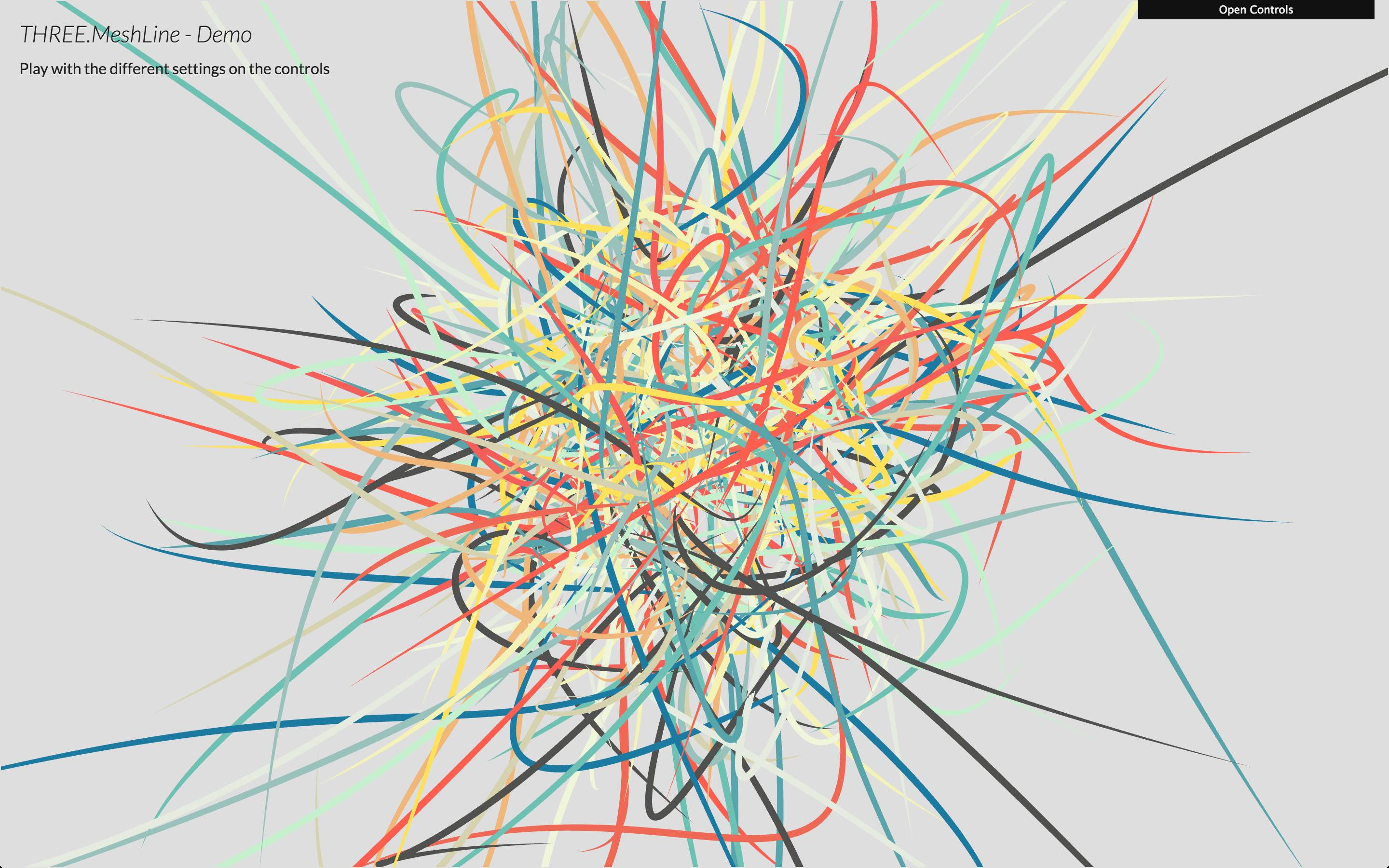• 送點對進去後畫出線條
• 可以調整寬度
• 可以調整顏色

## 解決方法：用點形成面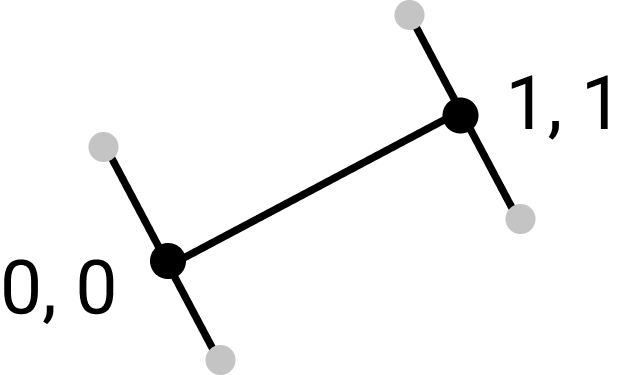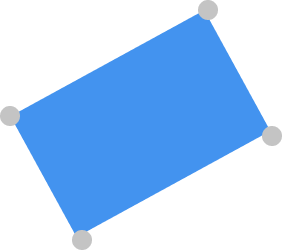## 實作

const indices = [0, 1, 2, 2, 1, 3]; // 告訴 webGL 存取頂點的順序
const indexBuffer = gl.createBuffer();
gl.bindBuffer(gl.ELEMENT_ARRAY_BUFFER, indexBuffer);
gl.bufferData(gl.ELEMENT_ARRAY_BUFFER, new Uint8Array(indices), gl.STATIC_DRAW);

## 方法一：對每個點求法線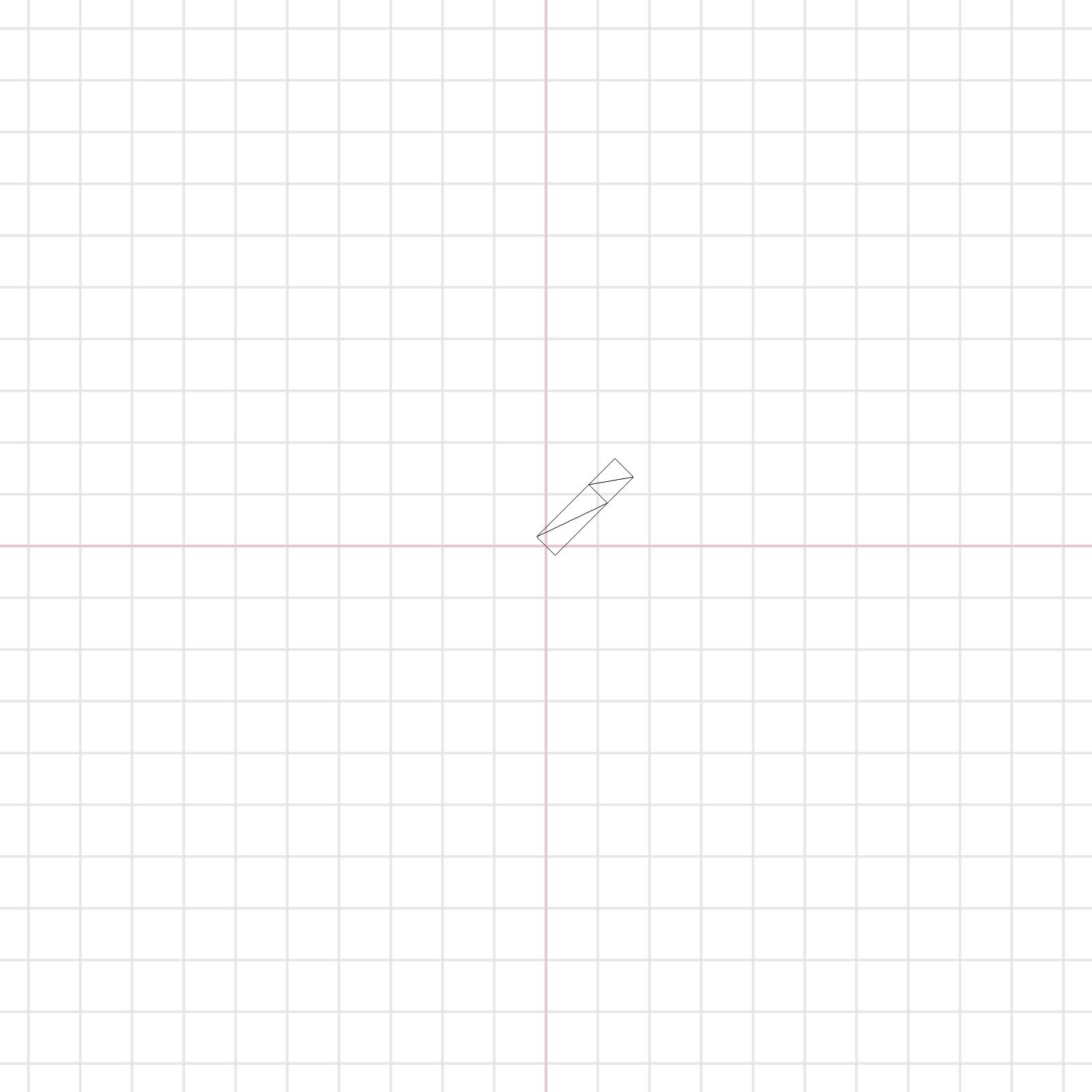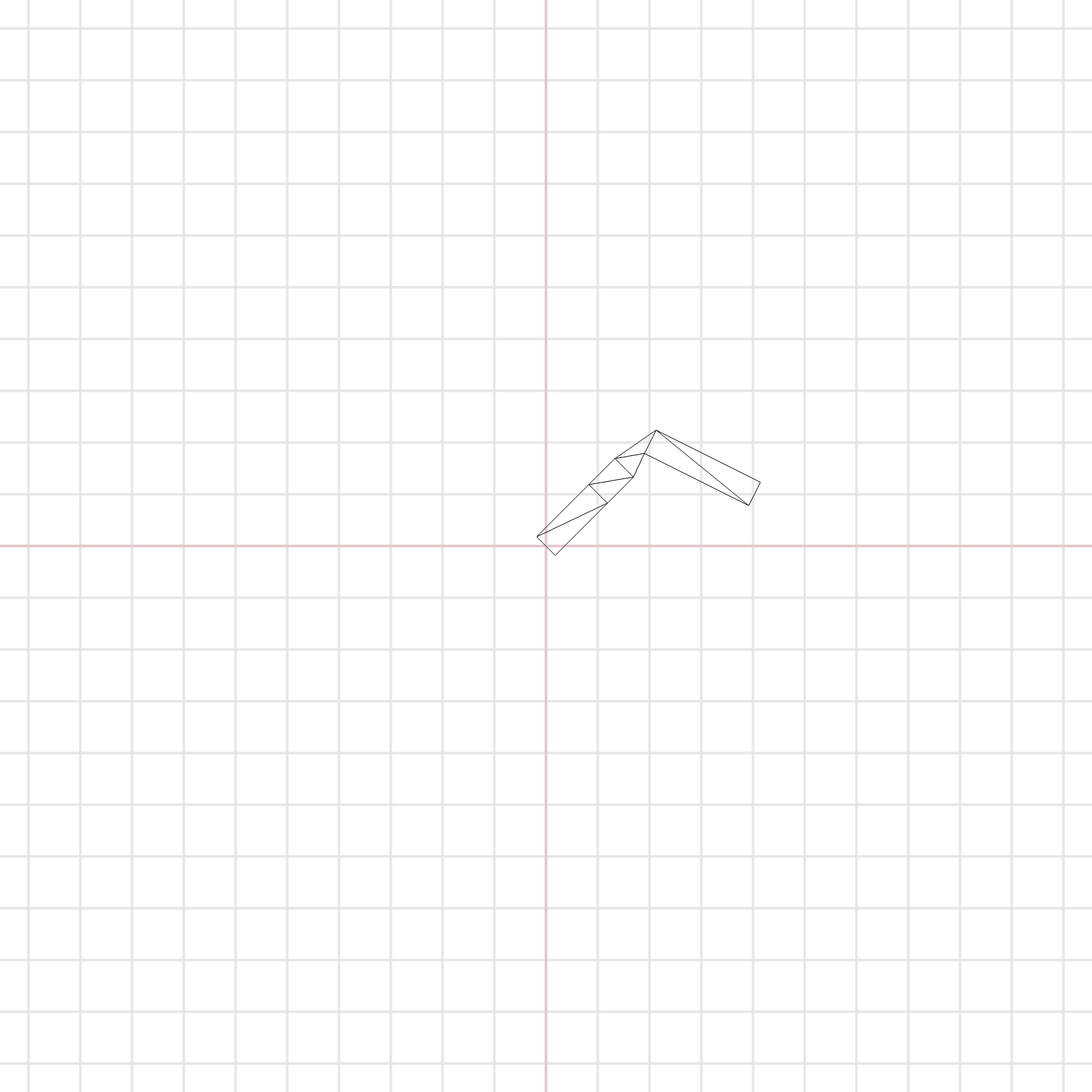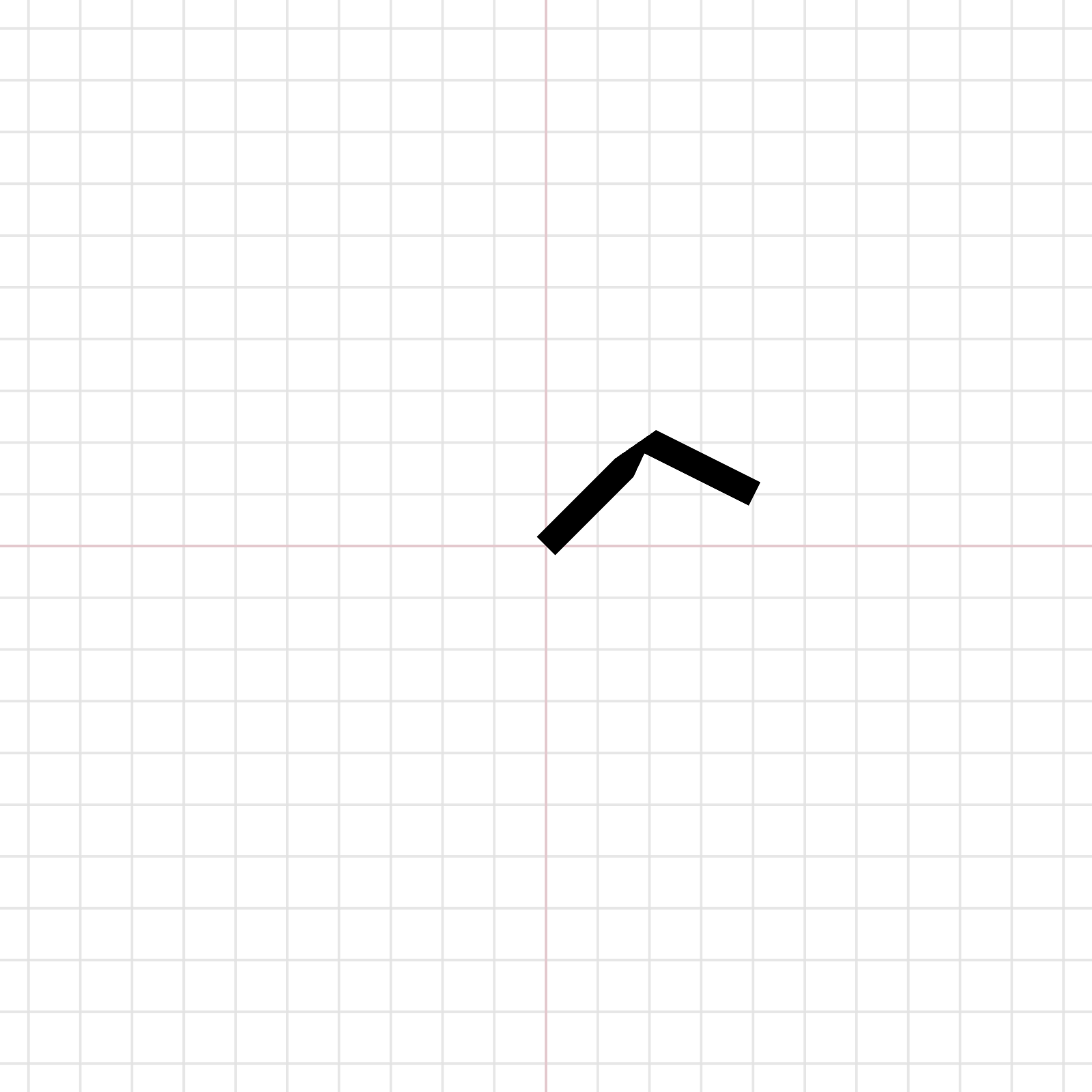## 轉折處處理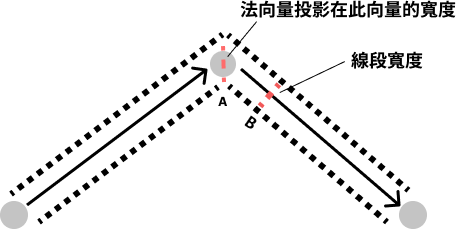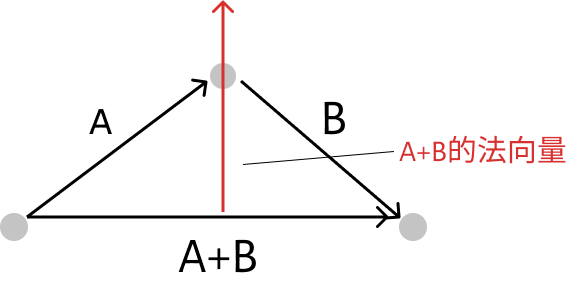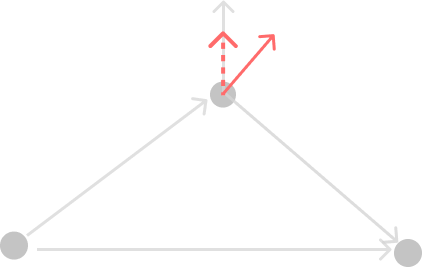• 求這個點與上個點的向量與下一個點的向量，假設分別為向量 A 與 B
• 計算 A 的法向量與 A+B 的法向量
• 計算 A+B 的法向量投影在 A 向量的長度
• 將線段長度除掉投影後的長度
• 在上下分別取一點，長度會是線段長度除 2

uniform vec3 uColor;
uniform float uLineWidth;
attribute vec3 lineNormal;
varying vec3 vColor;

void main() {
float width = (uLineWidth / lineNormal.z / 2.0);
vec3 pos = vec3(position + vec3(lineNormal.xy, 0.0) * width);
gl_Position = projectionMatrix * modelViewMatrix * vec4(pos, 1.0);
vColor = uColor;
}

#### BufferGeometry

export default class MyLineGeometry extends BufferGeometry {
constructor(points) {
super()
const lineNormal = []
const vertices = []
const indices = []
const last = points[points.length - 1]
let currentIdx = 0

points.forEach((p, index) => {
if (index <= points.length - 2) {
indices[index * 6 + 0] = currentIdx
indices[index * 6 + 1] = currentIdx + 1
indices[index * 6 + 2] = currentIdx + 2
indices[index * 6 + 3] = currentIdx + 2
indices[index * 6 + 4] = currentIdx + 1
indices[index * 6 + 5] = currentIdx + 3
currentIdx += 2
} else if (points.length === 2 && index === 0) {
indices[index * 6 + 0] = currentIdx
indices[index * 6 + 1] = currentIdx + 1
indices[index * 6 + 2] = currentIdx + 2
indices[index * 6 + 3] = currentIdx + 2
indices[index * 6 + 4] = currentIdx + 1
indices[index * 6 + 5] = currentIdx + 3
currentIdx += 2
}

vertices.push(p, p, 0)
vertices.push(p, p, 0)
})

for (let i = 1; i < points.length; i++) {
const point = points[i]
const prev = points[i - 1]
const next = points[i + 1] || null

const a = new Vector2(point - prev, point - prev).normalize()
if (i === 1) { // first point
lineNormal.push(-a.y, a.x, 1)
lineNormal.push(-a.y, a.x, -1)
}

if (!next) {
lineNormal.push(-a.y, a.x, 1)
lineNormal.push(-a.y, a.x, -1)
} else {
const b = new Vector2(next - point, next - point).normalize().add(a)
const c = new Vector2(-b.y, b.x)
const projection = c.clone().dot(new Vector2(-a.y, a.x).normalize())
lineNormal.push(c.x, c.y, projection)
lineNormal.push(c.x, c.y, -projection)
}
}

this.setAttribute('position', new BufferAttribute(new Float32Array(vertices), 3));
this.setAttribute('lineNormal', new BufferAttribute(new Float32Array(lineNormal), 3)); // [x, y, projection]
this.setIndex(new BufferAttribute(new Uint16Array(indices), 1));
const indexAttr = this.getIndex()
this.position.needsUpdate = true;
indexAttr.needsUpdate = true;
}
}

varying vec3 vColor;
varying vec2 vUv;
void main() {
float dist = length(vUv - 0.5);
vec3 color = vColor;
if (dist > 0.1) {
color = smoothstep(dist - 0.02, dist, vUv.y) * color;
}
gl_FragColor = vec4(color, 1.0);
}

import { shaderMaterial } from "@react-three/drei";
import { Color, DoubleSide } from "three";

{
uColor: new Color(0.0, 0.0, 0.0, 1.0),
uLineWidth: 10,
},
(material) => material.side = DoubleSide
)

export default MyLineShaderMaterial;

## 展示成果

### 有轉折的線條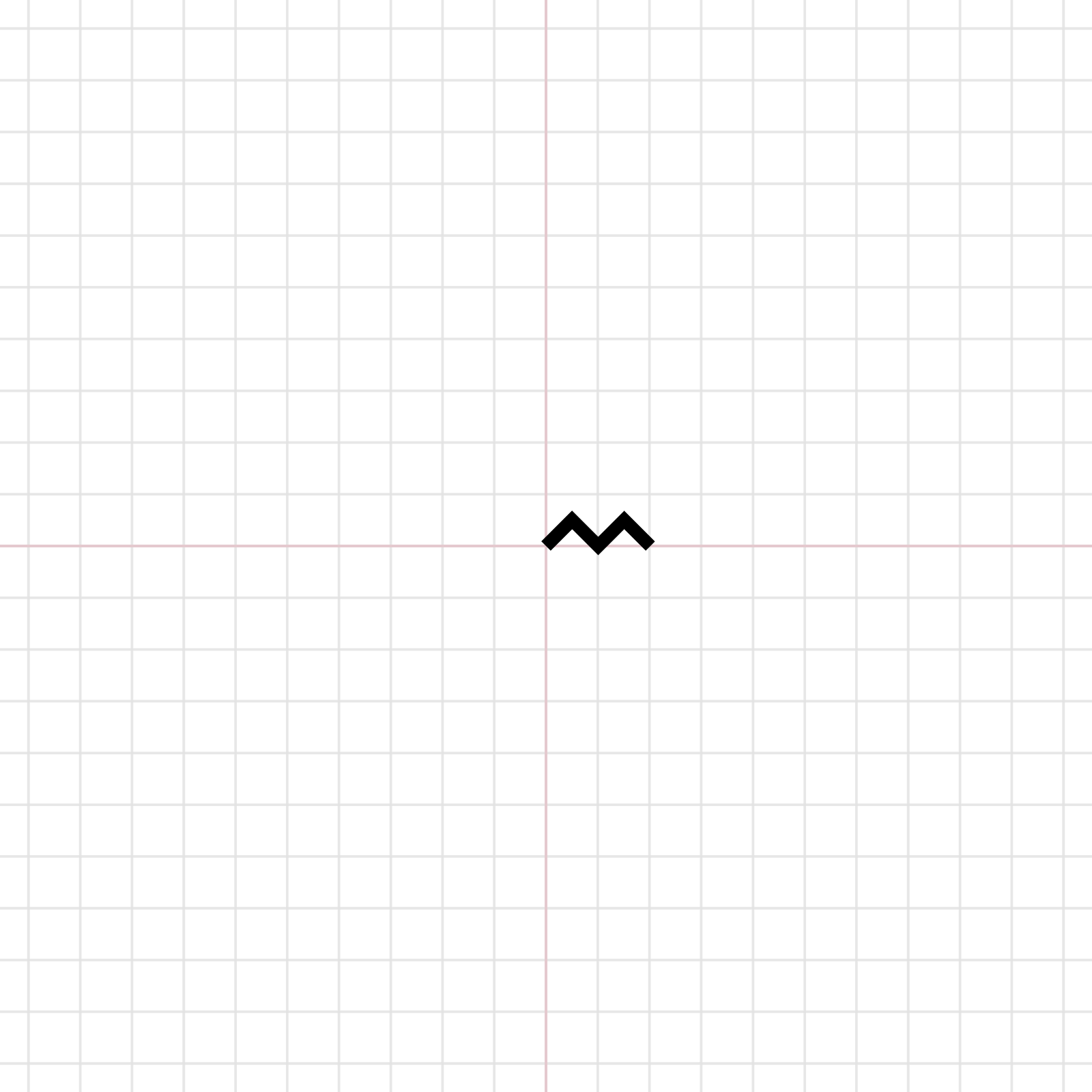### 正弦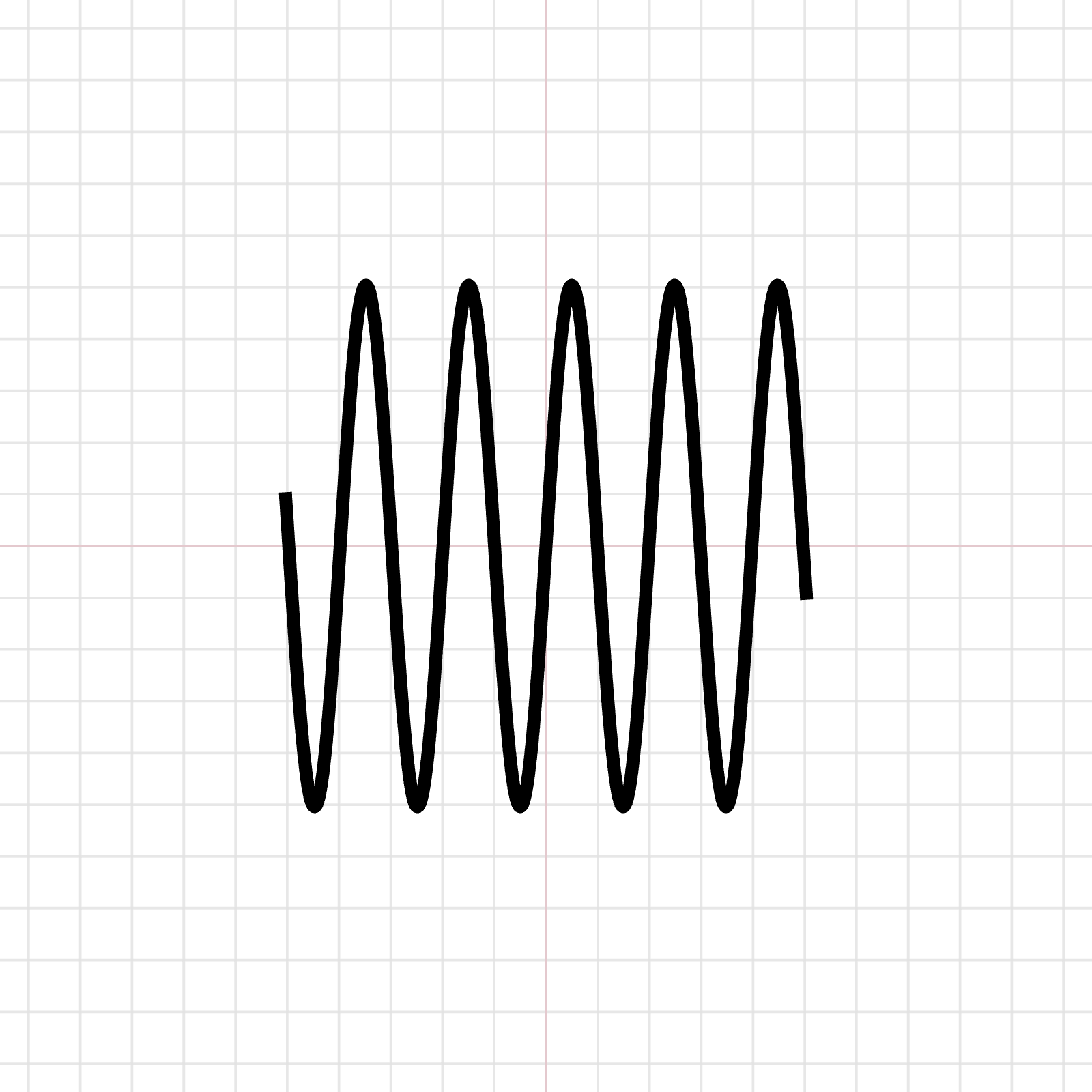## 下一個問題：去鋸齒化（anti-aliasing）• 直接用 texture mapping 處理
• 實作 Prefiltered line 處理

varying vec3 vColor;
varying vec2 vUv;
void main() {
float dist = length(vUv - 0.5);
vec3 color = vColor;
float progress = smoothstep(1.0 - 0.03, 1.0, 1.0 - dist);
if (dist > 0.9) {
vec3 col = mix(color, vec3(1, 1, 1), progress);
gl_FragColor = vec4(col, 1.0);
} else {
gl_FragColor = vec4(color, 1.0);
}
}

## 總結

• 線段可以做 round → 在轉折處需要另外設計頂點座標
• 每個線段有不同顏色
• 線段當中的粗細變化